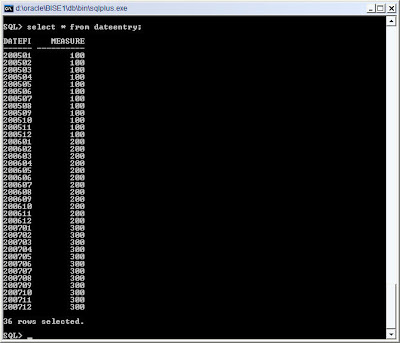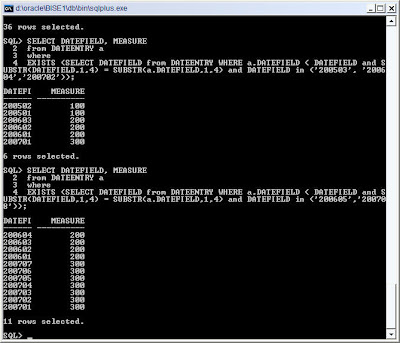# Archive for October 15th, 2007

## Oracle BI EE 10.1.3.3/2 – Variation of YTD function

Posted by Venkatakrishnan J on October 15, 2007

Another interesting question came up in the forums last week. Though the question was pretty straight forward, i thought of blogging about it here since it involves the use of evaluate function. The question was similar to the one below. I have rephrased it for clarity sake.

“We are in the process of creating some YTD reports wherein the users can choose mutiple date ranges of the format ‘yyyymm’. So, if a user chooses ‘200605’ and ‘200708’, then we need to produce an output which would be sum of all the data(measure of the report) from ‘200601’ to ‘200604’ and ‘200701’ and ‘200707’”

So basically, it is a modification of a simple YTD report. But what makes this interesting is that the months that user chooses can vary across years, as shown above in the question. So if a user chooses ‘200605’ and ‘200708’ our output should be a summation of our desired measure across the first 5 months in 2006 and the first 7 months in 2008. Now lets start approaching this problem from a plain sql point of view. Lets create a sample table called Dateentry.

CREATE TABLE DATEENTRY(DATEFIELD VARCHAR2(6), MEASURE NUMBER);For the sake of simplicity, i have specified the date fields as varchar. Also, note that we only have a month level granularity to keep it simple. To understand this better, i have the value of all the measures in the year 2005 as 100, 2006 as 200 and 2007 as 300. Now lets get the above report using a simple sql. The sql would look like this

SELECT DATEFIELD, MEASURE
from DATEENTRY a
where
EXISTS (SELECT DATEFIELD from DATEENTRY WHERE a.DATEFIELD < DATEFIELD and SUBSTR(DATEFIELD,1,4) = SUBSTR(a.DATEFIELD,1,4) and DATEFIELD in (‘200605′,’200708’))SELECT SUM(MEASURE)
from DATEENTRY a
where
EXISTS (SELECT DATEFIELD from DATEENTRY WHERE a.DATEFIELD < DATEFIELD and SUBSTR(DATEFIELD,1,4) = SUBSTR(a.DATEFIELD,1,4) and DATEFIELD in (‘200605′,’200708’))As you see above, this query basically involves a set by set comparison of the data and then applying filtering on top of that. Now lets see how to do this in OBI EE. Lets start with building a dashboard prompt. Remember, we cannot have a multi select dashboard prompt here since currently it cannot set presentation variables. We would have to go with a dashboard prompt wherein the users would have to enter the dates in comma seperated format.

In the dashboard prompt, we would set a presentation variable called “datefield”. Now lets create a function in the database which would basically use the above query to generate the sum of “Monthly YTD”. The function would look like this

CREATE OR REPLACE FUNCTION DATEFUN(FILTER IN VARCHAR2) RETURN NUMBER AS
v_sum number;
BEGIN
EXECUTE IMMEDIATE ‘SELECT SUM(MEASURE) from dateentry a
where
exists (select datefield from dateentry where a.datefield < datefield and SUBSTR(DATEFIELD,1,4) = SUBSTR(a.DATEFIELD,1,4) and datefield in ‘||'(‘||FILTER||’)’||’)’ into v_sum;
RETURN v_SUM;
end DATEFUN;

Now lets create a report which would basically call this function and pass the presentation variable “datefield” to this function. We will be using the EVALUATE function.

Remember, to encapsulate the presentation variable with IFNULL clause. If you do not, then you will receive some socket errors and strangely your BI Server would stop immediately. I am not sure whether this is a bug but the ifnull clause will make it work. Once this done, lets test it out by building a simple dashboard with the dashboard prompt and the report.

I am not sure whether this can be implemented out of the box using BI EE since it involves a set by set comparison. If any one has any other ideas, feel free to let me know.

Posted in All Posts, OBI EE Plus | 1 Comment »